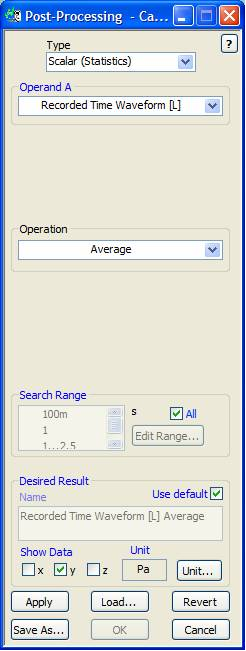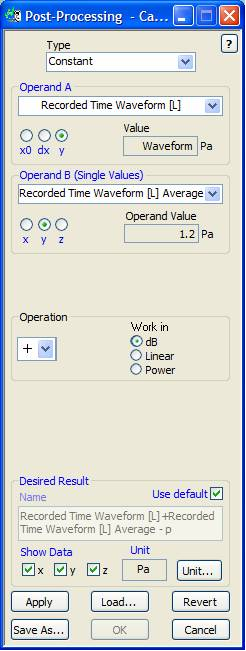# How Do I Remove a DC Offset From an Acquired Waveform?

To remove the DC offset from a waveform, first you need to estimate the DC level of the waveform by calculating the Average in a Post-Processing step. Then you can subtract the DC level from the waveform using a Constant Post-Processing step.

1. Estimate the DC level of the waveform by taking the Average in a Post-Processing step.2. Then Subtract the DC level from the waveform using Post-Processing > Constant.